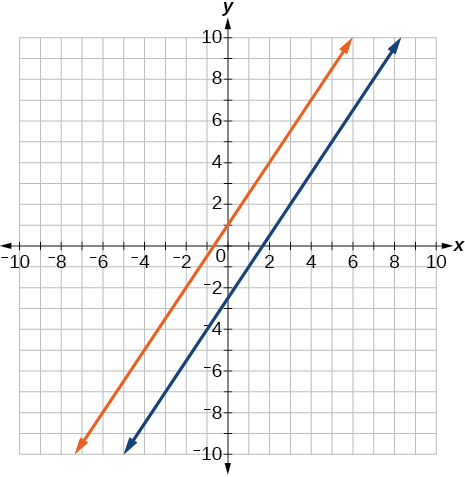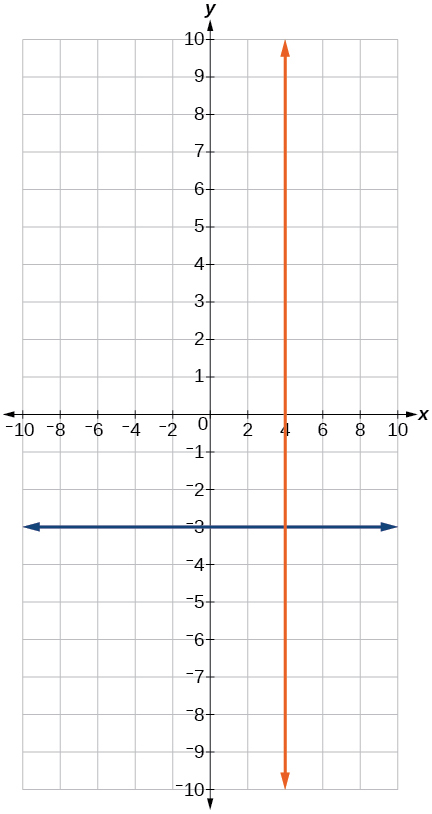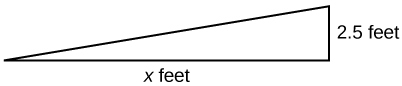# 2.2 Linear equations in one variable  (Page 8/15)

 Page 8 / 15

$\frac{x+2}{4}-\frac{x-1}{3}=2$

$x=-14$

For the following exercises, solve each rational equation for $\text{\hspace{0.17em}}x.\text{\hspace{0.17em}}$ State all x -values that are excluded from the solution set.

$\frac{3}{x}-\frac{1}{3}=\frac{1}{6}$

$2-\frac{3}{x+4}=\frac{x+2}{x+4}$

$x\ne -4;$ $x=-3$

$\frac{3}{x-2}=\frac{1}{x-1}+\frac{7}{\left(x-1\right)\left(x-2\right)}$

$\frac{3x}{x-1}+2=\frac{3}{x-1}$

$x\ne 1;$ when we solve this we get $\text{\hspace{0.17em}}x=1,$ which is excluded, therefore NO solution

$\frac{5}{x+1}+\frac{1}{x-3}=\frac{-6}{{x}^{2}-2x-3}$

$\frac{1}{x}=\frac{1}{5}+\frac{3}{2x}$

$x\ne 0;$ $x=\frac{-5}{2}$

For the following exercises, find the equation of the line using the point-slope formula.

Write all the final equations using the slope-intercept form.

$\left(0,3\right)\text{\hspace{0.17em}}$ with a slope of $\text{\hspace{0.17em}}\frac{2}{3}$

$\left(1,2\right)\text{\hspace{0.17em}}$ with a slope of $\text{\hspace{0.17em}}\frac{-4}{5}$

$y=\frac{-4}{5}x+\frac{14}{5}$

x -intercept is 1, and $\text{\hspace{0.17em}}\left(-2,6\right)$

y -intercept is 2, and $\text{\hspace{0.17em}}\left(4,-1\right)$

$y=\frac{-3}{4}x+2$

$\left(-3,10\right)\text{\hspace{0.17em}}$ and $\text{\hspace{0.17em}}\left(5,-6\right)$

$y=\frac{1}{2}x+\frac{5}{2}$

parallel to $\text{\hspace{0.17em}}y=2x+5\text{\hspace{0.17em}}$ and passes through the point $\text{\hspace{0.17em}}\left(4,3\right)$

perpendicular to $\text{\hspace{0.17em}}\text{3}y=x-4\text{\hspace{0.17em}}$ and passes through the point $\text{\hspace{0.17em}}\left(-2,1\right)$ .

$y=-3x-5$

For the following exercises, find the equation of the line using the given information.

$\left(-2,0\right)\text{\hspace{0.17em}}$ and $\text{\hspace{0.17em}}\left(-2,5\right)$

$\left(1,7\right)\text{\hspace{0.17em}}$ and $\text{\hspace{0.17em}}\left(3,7\right)$

$y=7$

The slope is undefined and it passes through the point $\text{\hspace{0.17em}}\left(2,3\right).$

The slope equals zero and it passes through the point $\text{\hspace{0.17em}}\left(1,-4\right).$

$y=-4$

The slope is $\text{\hspace{0.17em}}\frac{3}{4}\text{\hspace{0.17em}}$ and it passes through the point $\text{\hspace{0.17em}}\text{(1,4)}\text{.}$

$\left(-1,3\right)\text{\hspace{0.17em}}$ and $\text{\hspace{0.17em}}\left(4,-5\right)$

$8x+5y=7$

## Graphical

For the following exercises, graph the pair of equations on the same axes, and state whether they are parallel, perpendicular, or neither.

$\begin{array}{l}\\ \begin{array}{l}y=2x+7\hfill \\ y=\frac{-1}{2}x-4\hfill \end{array}\end{array}$

$\begin{array}{l}3x-2y=5\hfill \\ 6y-9x=6\hfill \end{array}$Parallel

$\begin{array}{l}y=\frac{3x+1}{4}\hfill \\ y=3x+2\hfill \end{array}$

$\begin{array}{l}x=4\\ y=-3\end{array}$Perpendicular

## Numeric

For the following exercises, find the slope of the line that passes through the given points.

$\left(5,4\right)\text{\hspace{0.17em}}$ and $\text{\hspace{0.17em}}\left(7,9\right)$

$\left(-3,2\right)\text{\hspace{0.17em}}$ and $\text{\hspace{0.17em}}\left(4,-7\right)$

$m=\frac{-9}{7}$

$\left(-5,4\right)\text{\hspace{0.17em}}$ and $\text{\hspace{0.17em}}\left(2,4\right)$

$\left(-1,-2\right)\text{\hspace{0.17em}}$ and $\text{\hspace{0.17em}}\left(3,4\right)$

$m=\frac{3}{2}$

$\text{\hspace{0.17em}}\left(3,-2\right)$ and $\text{\hspace{0.17em}}\left(3,-2\right)$

For the following exercises, find the slope of the lines that pass through each pair of points and determine whether the lines are parallel or perpendicular.

${m}_{1}=\frac{-1}{3},\text{ }{m}_{2}=3;\text{ }\text{Perpendicular}\text{.}$

## Technology

For the following exercises, express the equations in slope intercept form (rounding each number to the thousandths place). Enter this into a graphing calculator as Y1, then adjust the ymin and ymax values for your window to include where the y -intercept occurs. State your ymin and ymax values.

$0.537x-2.19y=100$

$y=0.245x-45.662.\text{\hspace{0.17em}}$ Answers may vary.

$4,500x-200y=9,528$

$\frac{200-30y}{x}=70$

$y=-2.333x+6.667.\text{\hspace{0.17em}}$ Answers may vary.

## Extensions

Starting with the point-slope formula $\text{\hspace{0.17em}}y-{y}_{1}=m\left(x-{x}_{1}\right),$ solve this expression for $\text{\hspace{0.17em}}x\text{\hspace{0.17em}}$ in terms of $\text{\hspace{0.17em}}{x}_{1},y,{y}_{1},$ and $\text{\hspace{0.17em}}m.$

Starting with the standard form of an equation solve this expression for y in terms of $\text{\hspace{0.17em}}A,B,C,\text{\hspace{0.17em}}$ and $\text{\hspace{0.17em}}x.\text{\hspace{0.17em}}$ Then put the expression in slope-intercept form.

$y=\frac{-A}{B}x+\frac{C}{B}$

Use the above derived formula to put the following standard equation in slope intercept form: $\text{\hspace{0.17em}}7x-5y=25.$

Given that the following coordinates are the vertices of a rectangle, prove that this truly is a rectangle by showing the slopes of the sides that meet are perpendicular.

$\left(-1,1\right),\left(2,0\right),\left(3,3\right)\text{,}$ and $\text{\hspace{0.17em}}\left(0,4\right)$

Yes they are perpendicular.

Find the slopes of the diagonals in the previous exercise. Are they perpendicular?

## Real-world applications

The slope for a wheelchair ramp for a home has to be $\text{\hspace{0.17em}}\frac{1}{12}.\text{\hspace{0.17em}}$ If the vertical distance from the ground to the door bottom is 2.5 ft, find the distance the ramp has to extend from the home in order to comply with the needed slope.30 ft

If the profit equation for a small business selling $\text{\hspace{0.17em}}x\text{\hspace{0.17em}}$ number of item one and $\text{\hspace{0.17em}}y\text{\hspace{0.17em}}$ number of item two is $\text{\hspace{0.17em}}p=3x+4y,$ find the $\text{\hspace{0.17em}}y\text{\hspace{0.17em}}$ value when

For the following exercises, use this scenario: The cost of renting a car is $45/wk plus$0.25/mi traveled during that week. An equation to represent the cost would be $\text{\hspace{0.17em}}y=45+.25x,$ where $\text{\hspace{0.17em}}x\text{\hspace{0.17em}}$ is the number of miles traveled.

What is your cost if you travel 50 mi?

$57.50 If your cost were $\text{\hspace{0.17em}}\text{}63.75,$ how many miles were you charged for traveling? Suppose you have a maximum of$100 to spend for the car rental. What would be the maximum number of miles you could travel?

220 mi

find the value of 2x=32
divide by 2 on each side of the equal sign to solve for x
corri
use the y -intercept and slope to sketch the graph of the equation y=6x
how do we prove the quadratic formular
hello, if you have a question about Algebra 2. I may be able to help. I am an Algebra 2 Teacher
thank you help me with how to prove the quadratic equation
Seidu
may God blessed u for that. Please I want u to help me in sets.
Opoku
what is math number
4
Trista
x-2y+3z=-3 2x-y+z=7 -x+3y-z=6
Need help solving this problem (2/7)^-2
x+2y-z=7
Sidiki
what is the coefficient of -4×
-1
Shedrak
the operation * is x * y =x + y/ 1+(x × y) show if the operation is commutative if x × y is not equal to -1
An investment account was opened with an initial deposit of \$9,600 and earns 7.4% interest, compounded continuously. How much will the account be worth after 15 years?
lim x to infinity e^1-e^-1/log(1+x)
given eccentricity and a point find the equiation
12, 17, 22.... 25th term
12, 17, 22.... 25th term
Akash
College algebra is really hard?
Absolutely, for me. My problems with math started in First grade...involving a nun Sister Anastasia, bad vision, talking & getting expelled from Catholic school. When it comes to math I just can't focus and all I can hear is our family silverware banging and clanging on the pink Formica table.
Carole
I'm 13 and I understand it great
AJ
I am 1 year old but I can do it! 1+1=2 proof very hard for me though.
Atone
Not really they are just easy concepts which can be understood if you have great basics. I am 14 I understood them easily.
Vedant
hi vedant can u help me with some assignments
Solomon
find the 15th term of the geometric sequince whose first is 18 and last term of 387
I know this work
salmaByByBy RhodesByByBy Nick Swain